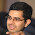## Thursday, 9 August 2012

### Four Digit Whole Number

Find a four digit number 'n' such that the last 4 digits of n^2 are same as that of n

#### 3 comments:

1.2.This comment has been removed by the author.

3.Clearly the last digit should be 0 or 1 or 5 or 6. It can't be 0 because that would lead to the number being 0000.

Notice that n^2 - n = n(n-1) is divisible by 10000 = 16*625.

If n ends in 1, n-1 ends in 0 which means 10000 divides n-1 which is not possible since n is four digit. So, this is not the case.

If n ends in 5, n = 625m and n= 16k+1 . Since 625%16 = 1, the least possible value of n > 625 is 625*17 which is not four digit. So, this is not the case.

If n ends in 6, n-1 ends in 5 i.e., n = 16k and n = 625m+1. The least possible value of m is 15 which makes n 9376.